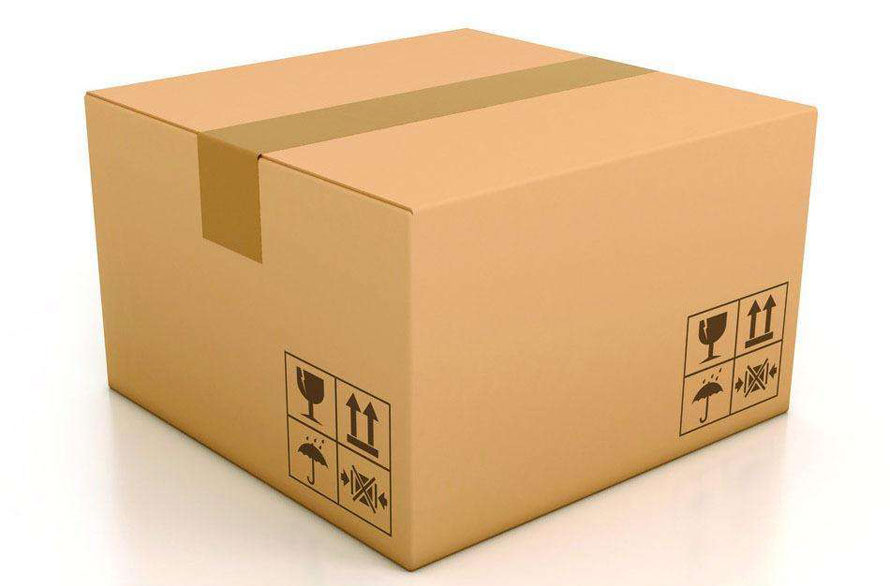Home>> Volumetric Weight >> Volumetric Weight air Freight

# Volumetric Weight air Freight And Chargeable Weight Calculation By Air

## Air Freight Volumetric Weight Formula

Iata air freight or international air cargo volumetric weight(dimension weight) formula have two simple ways:

• Air Freight Volumetric Weight(dimension weight)=(L*W*H)/6000=kilogram
L=Length, W=width, H=height
L/W/H are the package's longest size
measurements is use "CM" (centimetre)
inches to cm is (1:2.54)
• The second air cargo volumetric weight(dimension weight) Calculator is: 1 CBM (Cubic metre)=167 KG (kilogram).
When you know total CBM about your air cargo shipment you can use this air freight volumetric weight calculation formula easy get the total weight. On the air-bill show the total CBM also.

## Air Freight Chargeable Weight Calculation

When you use international air freight services, there have two weight, one is "volumetric weight", the other is "actual weight". Which is Chargeable Weight? General air cargo company will use which one is better.